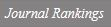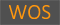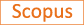On the rates of asymptotic regularity for some unbounded trajectories

Title
On the rates of asymptotic regularity for some unbounded trajectories
Authors
Kim I.
Ewha Authors
Issue Date
1999
Journal Title
Journal of Differential Equations
ISSN
0022-0396Citation
Journal of Differential Equations vol. 159, no. 2, pp. 307 - 320
Indexed
SCI; SCIE; SCOPUSDocument Type
Article
Abstract
Let T be a nonexpansive self-mapping of C where C is a nonempty closed convex subset of a Banach space E. We define T λ for 0&lt;λ&lt;1 by T λ=λT+(1-λ)I, where I is the identity operator on C, and denote x n=T n λx 0 where x 0∈C. Then the related initial value problem is du/dt=-(I-T)u(t) with u(0)=x 0∈C. The facts that

x n-Tx n

=O(1/n) as n→∞ and

u′(t)

=O(1/t) as t→∞ are known when C is bounded. In this paper we look for a rate of asymptotic regularity for

if

u(t)

=O(t α) where 0≤α≤1. We prove

=O(t -β) as t→∞, where α+2β=1 and obtain an estimate on

with the universal constant C α depending only on α. © 1999 Academic Press.
Appears in Collections:
자연과학대학 > 수학전공 > Journal papers
Files in This Item:
There are no files associated with this item.
Export
RIS (EndNote)
XLS (Excel)
XML